## Square Wells p.3

We have found a length scale L and an energy scale e, now we use these to transform Schrödinger's equation into dimensionless form. We make a dimensionless position: x'=x/L and a dimensionless energy: E'=E/e and plug those into Schrödinger's equation.This last equation should remind you of the (Newtonian) differential equation for a simple harmonic oscillator. (Note: derivatives with respect to x rather than time and E'-U'0 rather than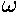2.)

We consider now two cases: (1) where the energy is more than the potential energy (so there is real kinetic energy---the classically allowed region) and (2) where the energy is less than the potential energy (so there is negative (?!) kinetic energy---the classically disallowed region)

### E > U0

Under these circumstances we can define a positive quantity k (we show below both dimensionless and dimensioned versions):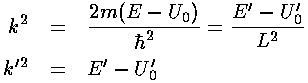(It turns out the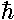k is the particle's momentum.)

Substituting k into the differential equation gives: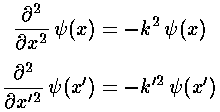with well known solutions:### E < U0

Under these circumstances we can define a positive quantity[Greek kappa] (we show below both dimensionless and dimensioned versions):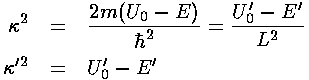Substituting k into the differential equation gives: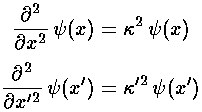with well known solutions: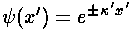We must now somehow connect the above two types of solutions. We begin with the easiest case:

### Infinite Square Well

In the case of the infinite square well regions of above type (2) are not just classically disallowed, they are infinitely disallowed. The wavefunction must be zero in such regions and since the wavefunction must be continuous, the wavefunction must be zero in the classically allowed region immediately adjacent to the infinitely disallowed region. Thus:(0)=(L)=0

Since cos(0)=1, the cosine solution violates the boundary condition, and cannot be the solution. sin(0)=0 automatically, but we must also have: sin(kL)=0 (or sin(k'1)=0). As is well known, sine has zeros at integer multiples of, so we must have:

kL=nFrom which we find the energy:The normalized wavefunctions are:If we use the symmetric origin choice ("walls" at ±a) we have the same equations for the eigenenergies and k but the form of the trigonometric functions changes between even and odd n:nE'
19.87
239.48
388.83
4157.91
5246.74
6355.31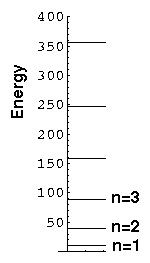Notice that we have a discrete set of energy levels, i.e., energy is quantized and the particle can have only the above energies. Quantum mechanics says energies between these levels will not be seen in nature. A discrete set of levels is expected if the particle is confined to a region.

Notice that the energy levels become further apart as you go up in energy. (Energy spacing (i.e., differences) are quite important as (1) that is what is observed in emitted photon energies and (2) that is what is related to the classical period.)

In the quantum fall, energy spacing decreased with increasing energy. In the quantum oscillator, the energy spacing was uniform. Here the energy spacings increase with energy. In a homework problem, you are to show that in the WKB approximation the energy spacing is proportional to the classical frequency, i.e., inversely proportional to the period T. SO I claim that in the square well the period decreases with increasing energy, and in the quantum fall the period increases with increasing energy. Is this true?